# High School Math : Understanding Polynomial Functions

## Example Questions

### Example Question #1 : Polynomial Functions

It took Jack 25 minutes to travel 14 miles, what was Jack's average speed in mph?

Possible Answers: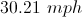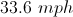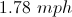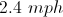Correct answer:Explanation: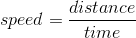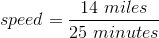* We have to change the time from minutes to hours, there are 60 minutes in one hour.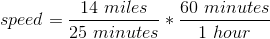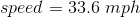### Example Question #2 : Polynomial Functions

Let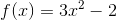and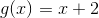.  Evaluate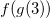.

Possible Answers: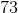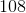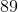Correct answer:Explanation:

Substituteinto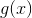, and then substitute the answer into.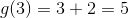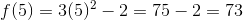### Example Question #3 : Polynomial Functions

Solve the following system of equations: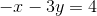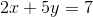Possible Answers: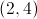Infinite solutions.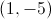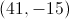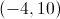Correct answer:Explanation:

We will solve this system of equations by Elimination.  Multiply both sides of the first equation by 2, to get: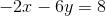Then add this new equation, to the second original equation, to get: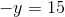or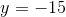Plugging this value ofback into the first original equation, gives: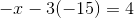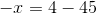or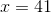### All High School Math Resources